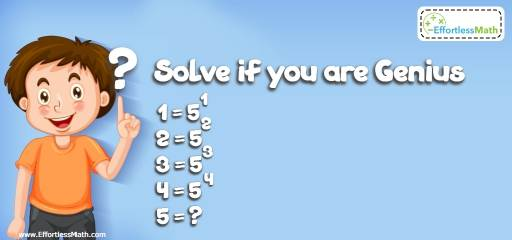# Number Properties Puzzle -Critical Thinking 2

Let's solve another critical challenge problem! This mathematical puzzle requires some kind of math to solve. Let's see how you or your kid can handle it! Time to challenge your brain!## Challenge:

$$1 = 5^1$$
$$2 = 5^2$$
$$3 = 5^3$$
$$4 = 5^4$$
$$5 = ?$$

### The Absolute Best Book to Challenge Your Smart Student!

For this question, don’t try to use the pattern! The first equation says that 1 equals to $$5^1$$, which equals to 5.
1 equals to 5. Therefore, 5 also equals to 1.
$$1 = 5^1$$
$$2 = 5^2$$
$$3 = 5^3$$
$$4 = 5^4$$
$$5=1$$

The Absolute Best Books to Ace Algebra

### What people say about "Number Properties Puzzle -Critical Thinking 2 - Effortless Math: We Help Students Learn to LOVE Mathematics"?

No one replied yet.

X
45% OFF

Limited time only!

Save Over 45%

SAVE $40 It was$89.99 now it is \$49.99